In :
%pylab inline

Welcome to pylab, a matplotlib-based Python environment [backend: module://IPython.zmq.pylab.backend_inline].

In :
import numpy as np
from pandas import *
from statsmodels.formula.api import logit
from statsmodels.nonparametric import KDE
import matplotlib.pyplot as plt
from patsy import dmatrix, dmatrices


# Logistic models of well switching in Bangladesh¶

Our data are information on about 3,000 respondent households in Bangladesh with wells having an unsafe amount of arsenic. The data record the amount of arsenic in the respondent's well, the distance to the nearest safe well (in meters), whether that respondent "switched" wells by using a neighbor's safe well instead of their own, as well as the respondent's years of education and a dummy variable indicating whether they belong to a community association.

Our goal is to model well-switching decision. Since it's a binary variable (1 = switch, 0 = no switch), we'll use logistic regression.

This analysis follows Gelman and Hill Data Analysis Using Regression and Multilevel/Hierarchical Models, chapter 5.4.

In :
df = read_csv('data/wells.dat', sep = ' ', header = 0, index_col = 0)

   switch  arsenic       dist  assoc  educ
1       1     2.36  16.826000      0     0
2       1     0.71  47.321999      0     0
3       0     2.07  20.966999      0    10
4       1     1.15  21.486000      0    12
5       1     1.10  40.874001      1    14


## Model 1: Distance to a safe well¶

For our first pass, we'll just use the distance to the nearest safe well. Since the distance is recorded in meters, and the effect of one meter is likely to be very small, we can get nicer model coefficients if we scale it. Instead of creating a new scaled variable, we'll just do it in the formula description using the I() function.

In :
model1 = logit('switch ~ I(dist/100.)', df = df).fit()
print model1.summary()

Optimization terminated successfully.
Current function value: 2038.118913
Iterations 4
Logit Regression Results
==============================================================================
Dep. Variable:                 switch   No. Observations:                 3020
Model:                          Logit   Df Residuals:                     3018
Method:                           MLE   Df Model:                            1
Date:                Sat, 22 Dec 2012   Pseudo R-squ.:                 0.01017
Time:                        13:05:25   Log-Likelihood:                -2038.1
converged:                       True   LL-Null:                       -2059.0
LLR p-value:                 9.798e-11
==================================================================================
coef    std err          z      P>|z|      [95.0% Conf. Int.]
----------------------------------------------------------------------------------
Intercept          0.6060      0.060     10.047      0.000         0.488     0.724
I(dist / 100.)    -0.6219      0.097     -6.383      0.000        -0.813    -0.431
==================================================================================


Let's plot this model. We'll want to jitter the switch data, since it's all 0/1 and will over-plot.

In :
def binary_jitter(x, jitter_amount = .05):
'''
Add jitter to a 0/1 vector of data for plotting.
'''
jitters = np.random.rand(*x.shape) * jitter_amount
x_jittered = x + np.where(x == 1, -1, 1) * jitters
return x_jittered

In :
dist_logit_par = model1.params['I(dist / 100.)']
plt.plot(df['dist'], binary_jitter(df['switch'], .1), '.', alpha = .1)
plt.plot(np.sort(df['dist']), model1.predict()[np.argsort(df['dist'])], lw = 2)
plt.ylabel('Switched Wells')
plt.xlabel('Distance from safe well (meters)')

Out:
<matplotlib.text.Text at 0x109862990>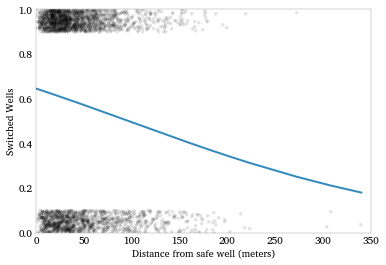Another way to look at this is to plot the densities of distance for switchers and non-switchers. We expect the distribution of switchers to have more mass over short distances and the distribution of non-switchers to have more mass over long distances.

In :
kde_sw = KDE(df['dist'][df['switch'] == 1])
kde_nosw = KDE(df['dist'][df['switch'] == 0])

kde_sw.fit()
kde_nosw.fit()

plt.plot(kde_sw.support, kde_sw.density, label = 'Switch')
plt.plot(kde_nosw.support, kde_nosw.density, color = 'red', label = 'No Switch')
plt.xlabel('Distance (meters)')
plt.legend(loc = 'best')

Out:
<matplotlib.legend.Legend at 0x109da8b50>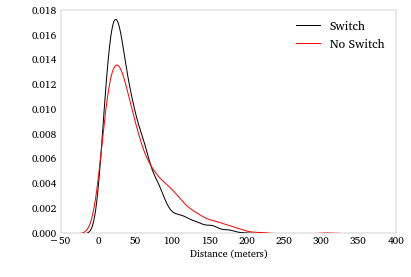## Model 2: Distance to a safe well and the arsenic level of own well¶

Next, let's add the arsenic level as a regressor. We'd expect respondents with higher arsenic levels to be more motivated to switch.

In :
model2 = logit('switch ~ I(dist / 100.) + arsenic', df = df).fit()
print model2.summary()

Optimization terminated successfully.
Current function value: 1965.334134
Iterations 5
Logit Regression Results
==============================================================================
Dep. Variable:                 switch   No. Observations:                 3020
Model:                          Logit   Df Residuals:                     3017
Method:                           MLE   Df Model:                            2
Date:                Sat, 22 Dec 2012   Pseudo R-squ.:                 0.04551
Time:                        13:05:29   Log-Likelihood:                -1965.3
converged:                       True   LL-Null:                       -2059.0
LLR p-value:                 1.995e-41
==================================================================================
coef    std err          z      P>|z|      [95.0% Conf. Int.]
----------------------------------------------------------------------------------
Intercept          0.0027      0.079      0.035      0.972        -0.153     0.158
I(dist / 100.)    -0.8966      0.104     -8.593      0.000        -1.101    -0.692
arsenic            0.4608      0.041     11.134      0.000         0.380     0.542
==================================================================================


Which is what we see. The coefficients are what we'd expect: the farther to a safe well, the less likely a respondent is to switch, but the higher the arsenic level in their own well, the more likely.

### Marginal effects¶

To see the effect of these on the probability of switching, let's calculate the marginal effects at the mean of the data.

In :
model2.margeff(at = 'mean')

Out:
array([-0.21806505,  0.11206108])

So, for the mean respondent, an increase of 100 meters to the nearest safe well is associated with a 22% lower probability of switching. But an increase of 1 in the arsenic level is associated with an 11% higher probability of switching.

### Class separability¶

To get a sense of how well this model might classify switchers and non-switchers, we can plot each class of respondent in (distance-arsenic)-space.

We don't see very clean separation, so we'd expect the model to have a fairly high error rate. But we do notice that the short-distance/high-arsenic region of the graph is mostly comprised switchers, and the long-distance/low-arsenic region is mostly comprised of non-switchers.

In :
logit_pars = model2.params
intercept = -logit_pars / logit_pars
slope = -logit_pars / logit_pars

dist_sw = df['dist'][df['switch'] == 1]
dist_nosw = df['dist'][df['switch'] == 0]
arsenic_sw = df['arsenic'][df['switch'] == 1]
arsenic_nosw = df['arsenic'][df['switch'] == 0]
plt.figure(figsize = (12, 8))
plt.plot(dist_sw, arsenic_sw, '.', mec = 'purple', mfc = 'None',
label = 'Switch')
plt.plot(dist_nosw, arsenic_nosw, '.', mec = 'orange', mfc = 'None',
label = 'No switch')
plt.plot(np.arange(0, 350, 1), intercept + slope * np.arange(0, 350, 1) / 100.,
'-k', label = 'Separating line')
plt.ylim(0, 10)
plt.xlabel('Distance to safe well (meters)')
plt.ylabel('Arsenic level')
plt.legend(loc = 'best')

Out:
<matplotlib.legend.Legend at 0x109f7cb10>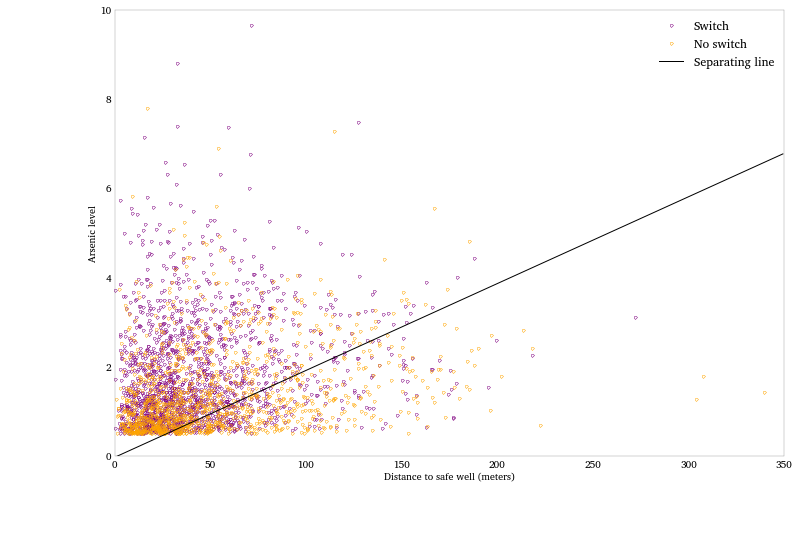## Model 3: Adding an interation¶

It's sensible that distance and arsenic would interact in the model. In other words, the effect of an 100 meters on your decision to switch would be affected by how much arsenic is in your well.

Again, we don't have to pre-compute an explicit interaction variable. We can just specify an interaction in the formula description using the : operator.

In :
model3 = logit('switch ~ I(dist / 100.) + arsenic + I(dist / 100.):arsenic',
df = df).fit()
print model3.summary()

Optimization terminated successfully.
Current function value: 1963.814202
Iterations 5
Logit Regression Results
==============================================================================
Dep. Variable:                 switch   No. Observations:                 3020
Model:                          Logit   Df Residuals:                     3016
Method:                           MLE   Df Model:                            3
Date:                Sat, 22 Dec 2012   Pseudo R-squ.:                 0.04625
Time:                        13:05:33   Log-Likelihood:                -1963.8
converged:                       True   LL-Null:                       -2059.0
LLR p-value:                 4.830e-41
==========================================================================================
coef    std err          z      P>|z|      [95.0% Conf. Int.]
------------------------------------------------------------------------------------------
Intercept                 -0.1479      0.118     -1.258      0.208        -0.378     0.083
I(dist / 100.)            -0.5772      0.209     -2.759      0.006        -0.987    -0.167
arsenic                    0.5560      0.069      8.021      0.000         0.420     0.692
I(dist / 100.):arsenic    -0.1789      0.102     -1.748      0.080        -0.379     0.022
==========================================================================================


The coefficient on the interaction is negative and significant. While we can't directly intepret its quantitative effect on switching, the qualitative interpretation gels with our intuition. Distance has a negative effect on switching, but this negative effect is reduced when arsenic levels are high. Alternatively, the arsenic level have a positive effect on switching, but this positive effect is reduced as distance to the nearest safe well increases.

## Model 4: Adding educuation, more interactions and centering variables¶

Respondents with more eduction might have a better understanding of the harmful effects of arsenic and therefore may be more likely to switch. Education is in years, so we'll scale it for more sensible coefficients. We'll also include interactions amongst all the regressors.

We're also going to center the variables, to help with interpretation of the coefficients. Once more, we can just do this in the formula, without pre-computing centered variables.

In :
model_form = ('switch ~ center(I(dist / 100.)) + center(arsenic) + ' +
'center(I(educ / 4.)) + ' +
'center(I(dist / 100.)) : center(arsenic) + ' +
'center(I(dist / 100.)) : center(I(educ / 4.)) + ' +
'center(arsenic) : center(I(educ / 4.))'
)
model4 = logit(model_form, df = df).fit()
print model4.summary()

Optimization terminated successfully.
Current function value: 1945.871775
Iterations 5
Logit Regression Results
==============================================================================
Dep. Variable:                 switch   No. Observations:                 3020
Model:                          Logit   Df Residuals:                     3013
Method:                           MLE   Df Model:                            6
Date:                Sat, 22 Dec 2012   Pseudo R-squ.:                 0.05497
Time:                        13:05:35   Log-Likelihood:                -1945.9
converged:                       True   LL-Null:                       -2059.0
LLR p-value:                 4.588e-46
===============================================================================================================
coef    std err          z      P>|z|      [95.0% Conf. Int.]
---------------------------------------------------------------------------------------------------------------
Intercept                                       0.3563      0.040      8.844      0.000         0.277     0.435
center(I(dist / 100.))                         -0.9029      0.107     -8.414      0.000        -1.113    -0.693
center(arsenic)                                 0.4950      0.043     11.497      0.000         0.411     0.579
center(I(educ / 4.))                            0.1850      0.039      4.720      0.000         0.108     0.262
center(I(dist / 100.)):center(arsenic)         -0.1177      0.104     -1.137      0.256        -0.321     0.085
center(I(dist / 100.)):center(I(educ / 4.))     0.3227      0.107      3.026      0.002         0.114     0.532
center(arsenic):center(I(educ / 4.))            0.0722      0.044      1.647      0.100        -0.014     0.158
===============================================================================================================


### Model assessment: Binned Residual plots¶

Plotting residuals to regressors can alert us to issues like nonlinearity or heteroskedasticity. Plotting raw residuals in a binary model isn't usually informative, so we do some smoothing. Here, we'll averaging the residuals within bins of the regressor. (A lowess or moving average might also work.)

In :
def bin_residuals(resid, var, bins):
'''
Compute average residuals within bins of a variable.

Returns a dataframe indexed by the bins, with the bin midpoint,
the residual average within the bin, and the confidence interval
bounds.
'''
resid_df = DataFrame({'var': var, 'resid': resid})
resid_df['bins'] = qcut(var, bins)
bin_group = resid_df.groupby('bins')
bin_df = bin_group['var', 'resid'].mean()
bin_df['count'] = bin_group['resid'].count()
bin_df['lower_ci'] = -2 * (bin_group['resid'].std() /
np.sqrt(bin_group['resid'].count()))
bin_df['upper_ci'] =  2 * (bin_group['resid'].std() /
np.sqrt(bin_df['count']))
bin_df = bin_df.sort('var')
return(bin_df)

def plot_binned_residuals(bin_df):
'''
Plotted binned residual averages and confidence intervals.
'''
plt.plot(bin_df['var'], bin_df['resid'], '.')
plt.plot(bin_df['var'], bin_df['lower_ci'], '-r')
plt.plot(bin_df['var'], bin_df['upper_ci'], '-r')
plt.axhline(0, color = 'gray', lw = .5)

arsenic_resids = bin_residuals(model4.resid, df['arsenic'], 40)
dist_resids = bin_residuals(model4.resid, df['dist'], 40)
plt.figure(figsize = (12, 5))
plt.subplot(121)
plt.ylabel('Residual (bin avg.)')
plt.xlabel('Arsenic (bin avg.)')
plot_binned_residuals(arsenic_resids)
plt.subplot(122)
plot_binned_residuals(dist_resids)
plt.ylabel('Residual (bin avg.)')
plt.xlabel('Distance (bin avg.)')

Out:
<matplotlib.text.Text at 0x10ae3ef50>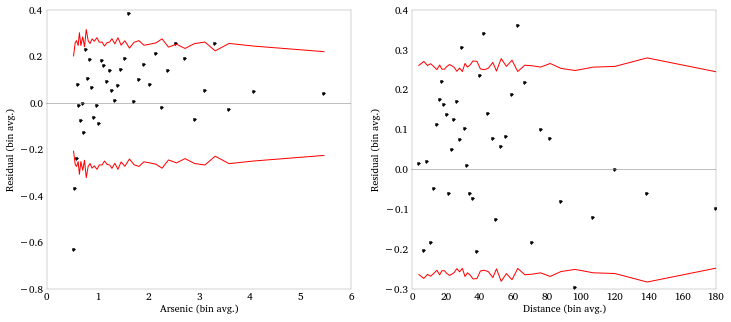## Model 5: log-scaling arsenic¶

The binned residual plot indicates some nonlinearity in the arsenic variable. Note how the model over-estimated for low arsenic and underestimates for high arsenic. This suggests a log transformation or something similar.

We can again do this transformation right in the formula.

In :
model_form = ('switch ~ center(I(dist / 100.)) + center(np.log(arsenic)) + ' +
'center(I(educ / 4.)) + ' +
'center(I(dist / 100.)) : center(np.log(arsenic)) + ' +
'center(I(dist / 100.)) : center(I(educ / 4.)) + ' +
'center(np.log(arsenic)) : center(I(educ / 4.))'
)

model5 = logit(model_form, df = df).fit()
print model5.summary()

Optimization terminated successfully.
Current function value: 1931.554102
Iterations 5
Logit Regression Results
==============================================================================
Dep. Variable:                 switch   No. Observations:                 3020
Model:                          Logit   Df Residuals:                     3013
Method:                           MLE   Df Model:                            6
Date:                Sat, 22 Dec 2012   Pseudo R-squ.:                 0.06192
Time:                        13:05:57   Log-Likelihood:                -1931.6
converged:                       True   LL-Null:                       -2059.0
LLR p-value:                 3.517e-52
==================================================================================================================
coef    std err          z      P>|z|      [95.0% Conf. Int.]
------------------------------------------------------------------------------------------------------------------
Intercept                                          0.3452      0.040      8.528      0.000         0.266     0.425
center(I(dist / 100.))                            -0.9796      0.111     -8.809      0.000        -1.197    -0.762
center(np.log(arsenic))                            0.9036      0.070     12.999      0.000         0.767     1.040
center(I(educ / 4.))                               0.1785      0.039      4.577      0.000         0.102     0.255
center(I(dist / 100.)):center(np.log(arsenic))    -0.1567      0.185     -0.846      0.397        -0.520     0.206
center(I(dist / 100.)):center(I(educ / 4.))        0.3384      0.108      3.141      0.002         0.127     0.550
center(np.log(arsenic)):center(I(educ / 4.))       0.0601      0.070      0.855      0.393        -0.078     0.198
==================================================================================================================


And the binned residual plot for arsenic now looks better.

In :
arsenic_resids = bin_residuals(model5.resid, df['arsenic'], 40)
dist_resids = bin_residuals(model5.resid, df['dist'], 40)
plt.figure(figsize = (12, 5))
plt.subplot(121)
plot_binned_residuals(arsenic_resids)
plt.ylabel('Residual (bin avg.)')
plt.xlabel('Arsenic (bin avg.)')
plt.subplot(122)
plot_binned_residuals(dist_resids)
plt.ylabel('Residual (bin avg.)')
plt.xlabel('Distance (bin avg.)')

Out:
<matplotlib.text.Text at 0x10aebb590>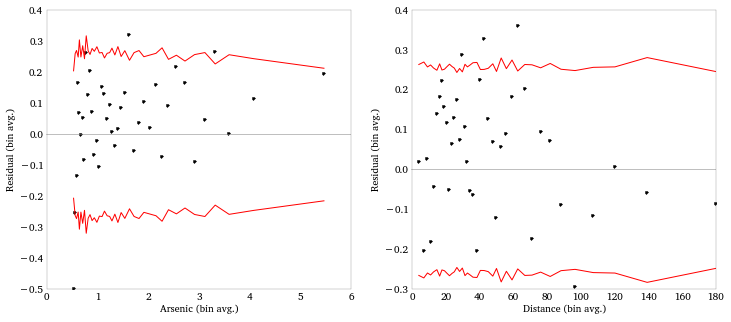### Model error rates¶

The pred_table() gives us a confusion matrix for the model. We can use this to compute the error rate of the model.

We should compare this to the null error rates, which comes from a model that just classifies everything as whatever the most prevalent response is. Here 58% of the respondents were switchers, so the null model just classifies everyone as a switcher, and therefore has an error rate of 42%.

In :
print model5.pred_table()
print 'Model Error rate: {0: 3.0%}'.format(
1 - np.diag(model5.pred_table()).sum() / model5.pred_table().sum())
print 'Null Error Rate: {0: 3.0%}'.format(
1 - df['switch'].mean())

[[  568.   715.]
[  387.  1350.]]
Model Error rate:  36%
Null Error Rate:  42%